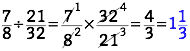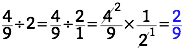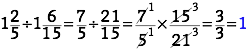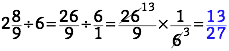# Doing the Flip

## OBJECTIVE: To learn how to divide fractions and mixed numbers

In previous lessons, you learned how to add, subtract, and multiply fractions and mixed numbers. Wearing Uniforms: https://www.geogebra.org/m/ceyu3wpa Mixing 'Em Up: https://www.geogebra.org/m/hzx95nwa Reducing, Reusing, Recycling: https://www.geogebra.org/m/gcxtdncg In this lesson, you're going to learn how to divide fractions and mixed numbers. DIVISION of FRACTIONS follows the exact same steps as MULTIPLICATION of FRACTIONS with one major difference. The first step in division involves FLIPPING (inverting) the SECOND FRACTION after which, the operation proceeds as in MULTIPLICATION of FRACTIONS. Examples:In DIVISION of MIXED NUMBERS, the first step involves CONVERTING the MIXED NUMBER into an IMPROPER FRACTION, after which the operation proceeds as in DIVISION of FRACTIONS as outlined above. Examples:## The applet below gives you practice on dividing fractions and mixed numbers.

Select a category or a pair of categories (as the applet will allow). Then solve the problem mentally or on a separate sheet of paper and verify your answer by clicking Answer. The applet will give the correct answer. Repeat as many times as needed to master the concept. Make sure to work on all categories.

## In this lesson, you learned how to divide fractions and mixed numbers.

In future lessons, you'll learn how to work with other forms of showing the parts of a whole. Did you ENJOY today's lesson?